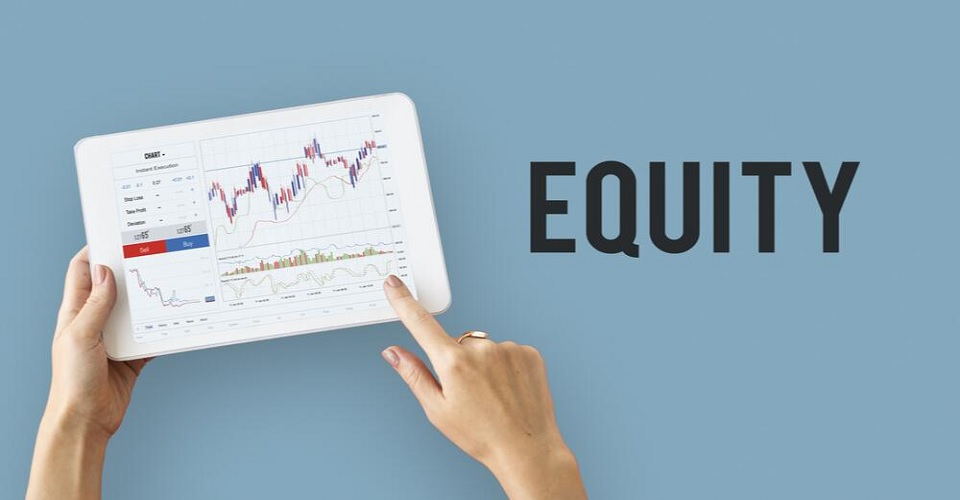# Blog

What is Equity in Forex?Forex equity describes the current value of one’s trading account. It is the degree of profit or loss that the account is sustaining when taking into account the open and closed positions at the time.

Naturally if there are no open positions, the equity will be the account’s balance. Calculating the equity when there are open positions requires the consideration of various parameters. These include the margin, the starting balance in the account and any unrealised profit or loss.

When open positions exist, the equity will be the sum of the account balance and the floating profits or losses.

It is important to understand the concept of forex equity, and to know the difference between the different types of equities, such as the available equity, and negative or positive equity. In a nutshell, the equity indicates the current account value and so any trader should be aware of it.

Many often think that equity is the margin. Often terms such as available margin, free margin, usable margin and floatable margin are encountered. The free margin is quite simply the forex equity as it refers to the total sum of funds that the trader has available to trade with.

The equity indicates the real time calculation of your profits and losses. The equity varies from one account to another as the total value of a trader’s forex account depends on his open and closed positions. When considering leverage, which many traders use, the equity on the account should be higher than the margin which is used for trades.

Another point worth mentioning while discussing the subject of forex equity is that there is the forex equity calculator – a helpful and easy to use tool that can help you in calculating the equity.